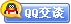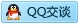##用户名 Email 自动登录 找回密码 密码 会员注册
 VIP会员，3年作业免费下 ！ 奥鹏作业，奥鹏毕业论文检测 新手作业下载教程，充值问题 没有找到答案，请在此处留言！ 2019年12月最新全国统考资料 投诉建议，加盟合作！奥鹏课程积分软件(ver:3.1)

# 北京地质大学2011春《高等数学》在线作业发表于 2011-6-20 17:46:15 | 显示全部楼层 |阅读模式一、单选题（共 10 道试题，共 40 分。） V 1.  下列函数中 （ ）是偶函数 A. x+sinx B. x+cosx C. xsinx D. xcosx       满分：4  分 2.  设f(x)=e^(2+x),则当△x→0时，f(x+△x)-f(x)→( ) A. △x B. e2+△x C. e2 D. 0       满分：4  分 3.  函数y=ln(x-1)在区间( )内有界。 A. (2,+∞) B. (1,+∞) C. (1,2) D. (2,3)       满分：4  分 4.  已知函数y= 2cos3x-5e^(2x), 则x=0时的微分dy=（ ） A. 10 B. 10dx C. -10 D. -10dx       满分：4  分 5.  函数在一点附近有界是函数在该点有极限的( ) A. 必要条件 B. 充分条件 C. 充分必要条件 D. 在一定条件下存在       满分：4  分 6.  如果函数f(x)的定义域为(0,1)则下列函数中，定义域为(-1,0)的为：（） A. f(1-x) B. f(1+x) C. f(sinx) D. f(cosx)       满分：4  分 7.  函数y=sin2x+cos4x的周期为 A. π B. 2π C. 3π D. 4π       满分：4  分 8.  函数y=|x-1|+2的极小值点是( ) A. 0 B. 1 C. 2 D. 3       满分：4  分 9.  函数y=cosx在[-1,+1]区间的拐点为 A. (0,0) B. (1,cos1) C. (-1,cos1) D. 无拐点       满分：4  分 10.  已知函数y= 2xsin3x-5e^(2x), 则x=0时的导数y'=（ ） A. 0 B. 10 C. -10 D. 1       满分：4  分 二、判断题（共 15 道试题，共 60 分。） V 1.  严格递增函数必有严格递增的反函数。（ ） A. 错误 B. 正确       满分：4  分 2.  设y=f(x)在区间[0,2008]上y′存在且恒大于0，则在区间[0,2008]上y是增函数。 A. 错误 B. 正确       满分：4  分 3.  若偶函数f(x)在x=0处的导数存在,则f'(0)的值=0 A. 错误 B. 正确       满分：4  分 4.  若函数y=lnx的x从1变到100,则自变量x的增量 Dx=99,函数增量Dy=ln100.（ ） A. 错误 B. 正确       满分：4  分 5.  在区间[0,1]上，函数y=x+tanx的导数恒大于0，所以是区间[0,1]上的增函数，从而最大值为1+tan1. A. 错误 B. 正确       满分：4  分 6.  初等函数都可导 A. 错误 B. 正确       满分：4  分 7.  对函数y=2008+x-sinx求导可得y′=1-cosx A. 错误 B. 正确       满分：4  分 8.  设{Xn}是无穷大量，{Yn}是有界数列，则{ XnYn }是无穷大量（ ） A. 错误 B. 正确       满分：4  分 9.  奇函数的图像关于 y 轴对称。 A. 错误 B. 正确       满分：4  分 10.  函数y=cos2x的4n阶导数为cos2x A. 错误 B. 正确       满分：4  分 11.  函数y=tan2x+cosx在定义域上既不是增函数也不是减函数 A. 错误 B. 正确       满分：4  分 12.  隐函数的导数表达式中不可含有y。（ ） A. 错误 B. 正确       满分：4  分 13.  函数定义的5个要素中，最重要的是掌握变量间的依存关系和定义域 A. 错误 B. 正确       满分：4  分 14.  导数又可视为因变量的微分和自变量微分的商 A. 错误 B. 正确       满分：4  分 15.  一元函数可导必连续，连续必可导。 A. 错误 B. 正确       满分：4  分 久爱奥鹏网：www.92open.com
 您需要登录后才可以回帖 登录 | 会员注册 本版积分规则 回帖并转播 回帖后跳转到最后一页客服一客服二客服三客服四微信客服扫一扫